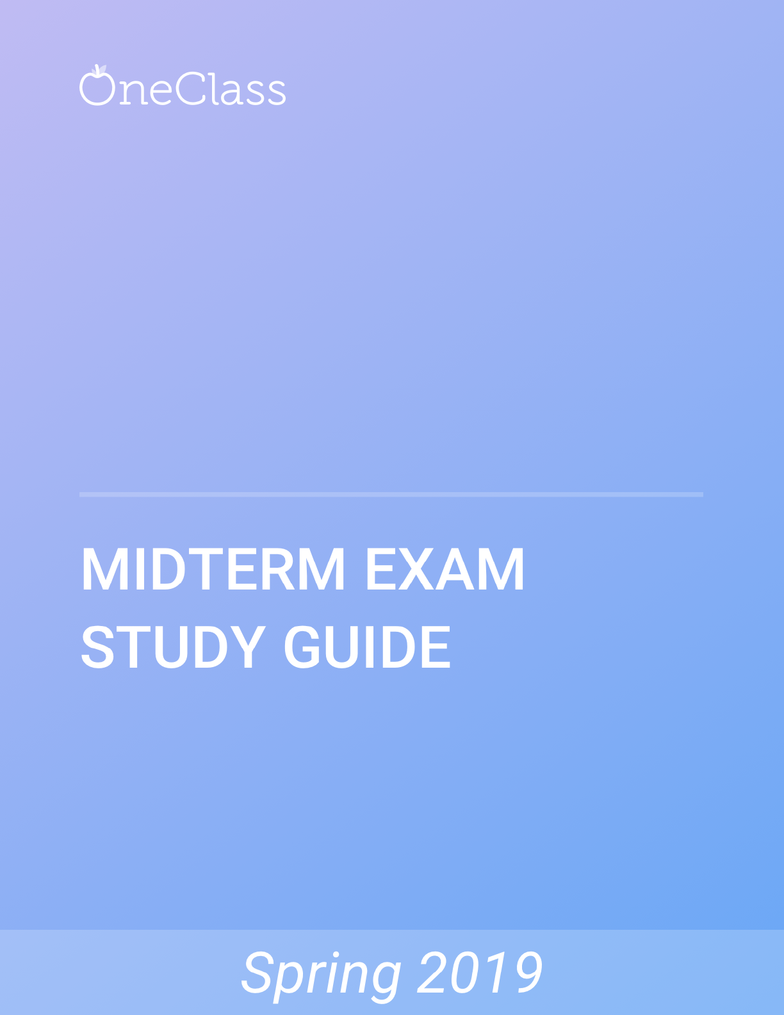# CHEM 230 Study Guide - Winter 2019, Comprehensive Midterm Notes - Watt, Torr, Thermometer

121 views17 pages
School
U of M
Department
Chemistry
Course
CHEM 230
ProfessorCHEM 230
Unlock document

This preview shows pages 1-3 of the document.
Unlock all 17 pages and 3 million more documents.Chemistry 230: Lecture 1
What is a gas?
We encounter a lot of gases in a lot of different places
Distributed uniformly throughout any container
Relatively low density and high volume
Ability to expand and contract with changes in pressure and temperature
Particles are constantly in motion and collide with other atoms or surfaces
Constant motion
Temperature is the average kinetic energy
All particles moving at different speeds in distribution average gives us the temperature
If two bodies are at different temperatures, heat will flow from warmer to cooler areas until
temperatures are the same and reaches equilibrium
The average kinetic energy is slightly higher than the most probably KE
Thermometer is used to measure temperature, it is a calibrated device thermal
Boiling Points/Different Temperature Scales
Boiling Point H2O
o 212°F
o 100°C
o 373.15 K
Freezing Point H2O
o 32°F
o 0°C
o 273.15 K
Freezing Point Salt H2O
o 0°F
o -18°C
o 255 K
Absolute Zero
o -460°F
o -273°C
o 0 K
Pressure
Atmospheric pressure (open system)
P = force/area = F/A = mass • acceleration / A = mg/A
P = mg/A
Higher pressure if we increase mass or decrease area
More pressure closer to earth’s surface (@ lower altitudes)
find more resources at oneclass.com
find more resources at oneclass.com
Unlock document

This preview shows pages 1-3 of the document.
Unlock all 17 pages and 3 million more documents.More pressure underwater (ears popping)
Less pressure up high
Use a mercury barometer to measure pressure
When pressures are equal, Patm = Pliquid = mg/A
Glass tube that is empy and inverted in mercury
o Mercury will rise up tube until pressure equilibrium is reached
o Similar to how a straw works
Pliquid = mg/A = dhAg/A = dhg
o *d and g are pretty constant!
Use liquid side to get the numer reading
o m/v = d m = dv m=dhA
Pressure Units
p = ma/A = kg•m/s2 / m2 = kg/ms2 = 1 Pa
1 bar = 1.00 • 105 Pa = 100 kPa
1 atm = 1.01 • 105 Pa = 101 kPa
1 atm = 760 mmHg = 760 torr
mmHg is pressure exerted by a column of mercury at 15°C in a standard gravitational field
Ideal Gas Law
P, V, n, R, T (closed system)
Gas molecules colliding with sides of container and each other always moving
Pressure = F/A
What would affect pressure?
o Decrease A to increase P
As you decrease V, pressure increases (inverse)
o Increase F to increase P
As you increase n, P increases (direct)
o Increase temperature, increase force, increase pressure (directly)
Focus on 2 variables at a time
Boyle’s Law
o Change volume and pressure (constant n, T)
o Isotherms (constant temperature)
o As pressure goes up, volume goes down
o Graph has lines that are each taken at different temperatures, but they show the
same relationship between P and V at different temperatures (constant!)
o Vary volume to measure pressure
Charles’ Law
o Temperature and volume change (constant P, n)
o All matter expands when heated, but gases have their degree of expansion is
independent of their composition
o When pressure is held constant, the volume of a gas changes 1/273 of its value for
every degree C°
find more resources at oneclass.com
find more resources at oneclass.com
Unlock document

This preview shows pages 1-3 of the document.
Unlock all 17 pages and 3 million more documents.o Use a container with pistons to find volume at different temperatures
o All lines intersect in on the negative x axis @ absolute zero
o T is x-axis and V is y-axis
o Change moles and volume (constant P, T)
o The same number of gas molecules will occupy the same volume under constant P
and T
o Does not matter what the gas is!
Summary of Gas Laws
Boyle’s: V 1/P
Charles: V T
V nT/P
PV=nRT
R is the universal gas constant
find more resources at oneclass.com
find more resources at oneclass.com
Unlock document

This preview shows pages 1-3 of the document.
Unlock all 17 pages and 3 million more documents.

## Document Summary

Temperature is the average kinetic energy: all particles moving at different speeds in distribution average gives us the temperature. Boiling points/different temperature scales: boiling point h2o, 212 f, 100 c, 373. 15 k, freezing point h2o, 32 f, 0 c, 273. 15 k, freezing point salt h2o, 0 f. Increase f to increase p: as you increase n, p increases (direct) Increase temperature, increase force, increase pressure (directly: focus on 2 variables at a time, boyle"s law, change volume and pressure (constant n, t) Summary of gas laws: boyle"s: v 1/p, charles: v t, avogadro: v n, v nt/p, pv=nrt, r is the universal gas constant. Vrms (root mean squared velocity: how fast are the gas molecules moving, where m = molecular weight of the gas, related to temperature and molecular weight, more massive particles at the same temperature move slower. Distribution and vrms vary with mw at the same t: higher mw, narrower distribution, lower vrms, compare noble gases.